### Fractions-Worksheets

CBSE Worksheet 01
Ch-7 Fractions

1. Which of the following is a smaller fraction?
(a) $\frac{4}{5}$ (b) $\frac{5}{3}$ (c) $\frac{5}{6}$ (d) $\frac{5}{2}$
2. Express as mixed fraction$\frac{16}{9}$.
1. $2\frac{7}{9}$
2. $1\frac{5}{9}$
3. $1\frac{7}{9}$
4. $2\frac{5}{9}$
3. What fraction of an hour is 45 minutes?
1. None of these
2. $\frac{3}{4}$
3. $\frac{1}{4}$
4. $\frac{1}{2}$
4. $\frac{17}{101}\mathrm{_}\mathrm{_}\mathrm{_}\mathrm{_}\frac{12}{101}$
(a) None of these (b) > (c) = (d) <
5. Write the fraction representing the shaded region in the given square.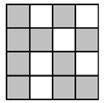1. $\frac{4}{16}$
2. $\frac{9}{16}$
3. $\frac{5}{16}$
4. $\frac{10}{16}$
6. Match the following:
Column IColumn II
(a)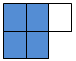(i) $\frac{5}{9}$
(b)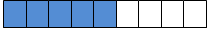(ii) $\frac{1}{2}$
(c)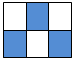(iii) $\frac{1}{3}$
(d)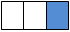(iv) $\frac{4}{5}$

7. Fill in the blanks:
There is a large box of 36 small square boxes.
1. $\frac{1}{2}$ of it is _____.
2. $\frac{2}{3}$ of it is _____.
3. If I make a bench of 20 small boxes, the fraction becomes _____.
4. _____ boxes are required if fraction is $\frac{5}{6}$.
8. State True or False:
1. In $\frac{3}{7}$, 3 is the part of whole.
2. On a number line, $\frac{2}{7}$ is to the right of zero.
3. $\frac{2}{5}$ is smaller than $\frac{1}{5}$.
4. $\frac{28}{45}$ and $\frac{3}{5}$ represent equivalent fractions.
9. Solve :$\frac{16}{5}-\phantom{\rule{thickmathspace}{0ex}}\frac{7}{5}$
10. Colour the part according to $\frac{3}{4}$.
11. Find the equivalent fraction $\frac{3}{5}$ having numerator 27.
12. Rewrite the fractions in the simplest form
1. $\frac{8}{6}$
2. $\frac{44}{72}$
13. Express the following as mixed fracton : $\frac{19}{6}$
14. Show $\frac{10}{10}$ on the number line.
15. Find the missing entries in the tables:
Fraction in standard formNumeratorDenominatorDiagrammatic Representation
$\frac{6}{7}$84a
b56
$\frac{9×2}{5×2}$c10
CBSE Worksheet 01
Ch-7 Fractions

1. $\frac{4}{5}$ Explanation: 4/5, 5/6 , 5/3 , 5/2 lcm of denominator(5,6,3,2)=30
$\frac{4}{5},\frac{5}{6},\frac{5}{3},\frac{5}{2}=\frac{24,25,50,75}{30}$ smaller fraction =$\frac{24}{30}$i.e $\frac{4}{5}$

1. $1\frac{7}{9}$ Explanation: $16÷9$ quotient is 1 which is a whole number and remainder is 7 which is a numerator of a proper fraction and denominator of proper fraction is 9 so mixed fraction = $1\frac{7}{9}$

1. $\frac{3}{4}$ Explanation: 1 hour = 60 minutes $\frac{45}{60}=\frac{45÷15}{60÷15}=\frac{3}{4}$
1. >
Explanation: denominator is 101 so $\frac{17}{101}>\frac{12}{101}$
1. $\frac{10}{16}$ Explanation: Total part = 16, shaded part = 10
Fraction = $\frac{10}{16}$
1. $\to$ (iv)
4 parts are shaded out of 5 parts.
Therefore, the shaded portion = $\frac{4}{5}$
2. $\to$ (i)
5 parts are shaded out of 9 parts.
Therefore, the shaded portion = $\frac{5}{9}$
3. $\to$ (ii)
3 parts are shaded out of 6 parts.
Therefore, the shaded portion = $\frac{3}{6}=\frac{3÷3}{6÷3}=\frac{1}{2}$
4. $\to$ (iii)
1 part is shaded out of 3 parts.
Therefore, the shaded portion = $\frac{1}{3}$
1. 18
$\frac{1}{2}$ of 36 boxes
$\frac{1}{2}×$ 36 = $\frac{36}{2}$ = 18 boxes.
2. 24
$\frac{2}{3}$ of 36 boxes
$\frac{2}{3}×$ 36 = $\frac{2×36}{3}$ = 2 x 12 = 24 boxes.
3. $\frac{5}{9}$
If a bench is made with 20 boxes out of 36 boxes, the fraction
$\frac{20}{36}=\frac{20÷4}{36÷4}=\frac{5}{9}$
4. 30
Let $x$ boxes out of 36 boxes makes fraction $\frac{5}{6}$
i.e., $\frac{x}{36}=\frac{5}{6}$
$x=\frac{5}{6}×36=\frac{5×36}{6}$ = 5 x 6 = 30.
Therefore, 30 boxes makes the fraction $\frac{5}{6}$
1. True
In the fraction, $\frac{3}{7}$, numerator is 3 and denominator is 7, which means that a unit has been divided into 7 equal parts out of which 3 parts have been taken into account.
2. True
All positive fractions are greater than zero and lies to the right of zero.
3. False
When denominators are same in two fractions, the fraction with the greater numerator is greater than the other fraction.
Here, in the frations $\frac{2}{5}and\frac{1}{5}$, denominators are same and in the numerators 2 and 1, 2 is greater than 1.
So, $\frac{2}{5}>\frac{1}{5}$
4. False
In the fractions, $\frac{28}{45}$ and $\frac{3}{5}$ comparing their cross products,
$28×5=140$ and $45×3=135$, we observe that they are not equal.
Hence, $\frac{28}{45}$ and $\frac{3}{5}$ are not equivalent fractions.
1. $\frac{16}{5}-\phantom{\rule{thickmathspace}{0ex}}\frac{7}{5}=\phantom{\rule{thickmathspace}{0ex}}\frac{16-7}{5}=\phantom{\rule{thickmathspace}{0ex}}\frac{9}{5}=\phantom{\rule{thickmathspace}{0ex}}1\frac{4}{5}\phantom{\rule{thickmathspace}{0ex}}$
2. $\frac{3}{4}$ means 3 parts out of 4 parts.
So, colour 3 parts out of 4 parts given.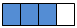3. $\frac{3}{5}=\frac{3×9}{5\phantom{\rule{thickmathspace}{0ex}}×\phantom{\rule{thickmathspace}{0ex}}9}=\frac{27}{45}$
1. $\frac{8}{6}=$ $\frac{8÷2}{6÷2}=\frac{4}{3}$
2. $\frac{44}{72}=$ $\frac{44÷2}{72÷2}=\frac{22÷2}{36÷2}=\frac{11}{18}$
4. $\frac{19}{6}$= 19 $÷$ 6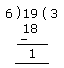$\therefore$$\frac{19}{6}=3\frac{1}{6}$
5. $\frac{10}{10}$ is 1 whole, which can be shown by the point 1.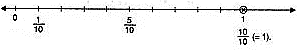6. Fraction in standard formNumeratorDenominatorDiagrammatic Representation
$\frac{6}{7}$84$\overline{)98}$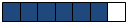$\overline{)\frac{5}{6}}$56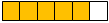$\frac{9}{5}=1\frac{4}{5}$$\overline{)18}$10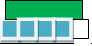a. $\frac{6}{7}$ = $\frac{6×14}{7×14}=\frac{84}{\overline{)98}}$ b. $\overline{)\frac{5}{6}}=\frac{5×1}{6×1}=\frac{5}{6}$ c.